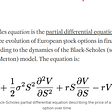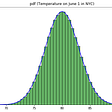# Time Complexity by Master Theorem

When working in big companies, it is required for a Software Developer that his code should have less time complexity and take less space of memory. Time complexity is the number of operations performed by an algorithm to complete the task. Whereas, space complexity is the amount of memory space taken by an algorithm in performing the same task. So, the efficiency of an algorithm depends on both the factors. Whether, a developer working on an app or website, efficiency of the application matters. Therefore, he should keep in mind these factors when improving or creating an algorithm. Hence, recurrence relations are used to analysis of time complexity of recursive functions. A recursive function is a function that repeatedly calls itself during its execution. For e.g. In Binary Search algorithm, the function repeatedly calls itself to find the element according to its position relative to middle element. While, recurrence relation is an equation that describes the function in terms of smaller inputs. For e.g., recurrence relation for Binary Search algorithm is T(n)= T(n/2) +c where c is constant. To calculate the time complexity of an algorithm that follows recurrence relation, there are four methods: Substitution method, Iteration method, Recursion Tree method and Master Theorem.

Master Theorem is used to find the running time of algorithms (divide and conquer) in terms of asymptotic notions(big-O). The approach was first presented by Jon Bentley, Dorothea Haken, and James B. Saxe in 1980, where it was described as a “unifying method”. Master Theorem is capable of solving the following type of recurrence relation.

T(n) = a*T(n/b) + f(n) where a & b are constants and a >= 1 and b>1.

Where n= size of the problem

a= number of subproblems in the recursion

n/b= size of each subproblem

f(n)= cost of work done outside the recursive calls and cost of combining them to get the solution.

Note: Not all algorithms can be solved using Master Theorem: -

1.When T(n) is not monotone. Ex: — T(n)=sin(n).

2.When f(n) is not polynomial. Ex: — T(n)=2T(n/2) +2**n (where n is power of 2).

How does this work?

Master theorem can be derived from recurrence tree relation. If we draw recurrence tree of T(n) = a*T(n/b) + f(n), then we can conclude that work done at leaf nodes is Θ(n**c), where c is log a to the base b and the height of the tree is log of n base b. The work done at the root is f(n).

In recurrence method, we calculate total work done. If work done at the leaves is polynomic -ally more then work done at leaves is dominant and result becomes work done at leaves. If work done at the roots and work done at leaves are equal, then work done becomes height multiplied by either of the work done. If work done at roots is more, then result becomes work done at the roots.

Rules and Applications:

Following is the extended Master Theorem which is used to calculate time complexity of algorithms that follows divide and conquer.

Where p is a real number and k>=0.

Then we consider following cases,

1. If a> b**k, then T(n) = θ(n**(log of a base b)), where k is power of b and log of a is of base b.

2. If a = b**k, then

(a) If p> -1, then T(n)= θ((n**log of a base b)* (log of n )**(p+1)), where p+1 is the power of log of n.

(b) If p= -1, then T(n) = θ((n**log of a base b) *loglog of n)

(c) If p< -1, then T(n)= θ(n**(log of a base b))

3. If a< b**k, then

(a) If p>= 0, then T(n) = θ((n**k)*(log of n)**p).

(b) If p< 0, then T(n)= θ(n**k).

## Let’s look at some of the examples:

1. Consider Binary search algorithm which has recurrence relation T(n)= T(n/2) + O(1) , where a=1,b=2,k=0 and p=0.

So, check if a>b**k or a=b**k or a<b**k. When putting values, we find that it satisfies a=b**k. Now check for p. p is equal to 0, hence it follows CASE2.1. i.e., T(n)= θ ((n** (log of a base b))* (log of n)**(p+1)). On putting the values, we get T(n)= θ(log of n).

Below is the link to the code of Binary search:

https://www.geeksforgeeks.org/binary-search/

Recurrence relation is a result because the input is divided by two each time function is called.

2. Consider second example of Merge sort algorithm which has recurrence relation T(n)= 2T(n/2) + O(n), where a=2, b=2, k=1 and p=0. Also, b**k =2 and a=2, so it satisfies CASE2.1. i.e., T(n)= θ ((n**(log of a base b))*(log of n)**(p+1)). On putting the values, we get T(n)= θ(n*log of n).

Below is the link to the code of Merge Sort:

https://www.geeksforgeeks.org/merge-sort/

Recurrence relation is a result as the input n is divided by two, dividing both the right and left arrays and then sorting them.

3. Now consider T(n)= (2**n)*T(n/2) + n**n, it cannot be solved by Masters Theorem as it is not in the form T(n)= a*T(n/b) + θ((n**k)*(log of n)**p).

4. Consider T(n) = 3T(n/2) + n**2, here a=3, b=2, k=2, p=0, b**k=2. So, a<b**k and p=0 (Case 3.1) ,putting all values T(n)=θ(n**2).

Usage of Master Theorem in Computer Science:

Master Theorem is used to analyze time complexity of recurrence functions. It makes calculation of finding time complexity easy and quick. It helps programmers to evaluate their code by finding time complexity and helps in correction or increase the performance of the code.

Conclusion:

In the analysis of algorithms, Master theorem provides an easy and one of the good ways to calculate time complexity of an algorithm. But it has some drawbacks, such as if a function is in the form of sin, cos, tan etc. or If a function is not polynomial for e.g., 2 to the power n, where n is input or If a is not constant, then Master Theorem is of no use in these cases. It is one of the good methods to solve recurrence function other than recursion tree, substitution method or iterative method.

--

--

--

## More from Kaustubh Joshi

Love podcasts or audiobooks? Learn on the go with our new app.

## Insight into Black Scholes Equation## What Sleeping Beauty Tells Us About Probabilities: Part I## The Central Limit Theorem, Simplified!## What’s Entropy? (Part-II)## Intuitions behind probability distributions## When Chance Meets Necessity: Part 1## What Color Is Math?## Watch collecting and the winner’s curse## The PactVC journey begins…## How HOTOVO’s Software Is Helping to Protect our Beautiful Planet## Amazon Cross-selling & Up-Selling## The Importance of Accuracy